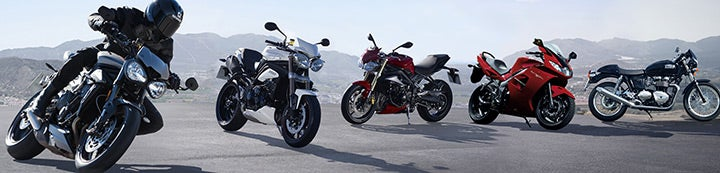1 - 10 of 10 Posts

#### barneys

·
##### Registered
Joined
·
622 Posts
Discussion Starter · ·
I have just bought a new torque wrench and have a question about the graduation markings on the barrel. One set is headed ft lbs Which I am Ok with. The other is headed M Kgs, which I although reversed, is Kilograms Metres. What I don't understand is above this scale it is marked (da N.m). What does that mean?

·
Joined
·
1,670 Posts
Neuton meters

·
Joined
·
3,701 Posts

#### barneys

·
##### Registered
Joined
·
622 Posts
Discussion Starter · ·
I realise that Nm is Newton Metres. What I can't understand is why the legend (da Nm) is immediately above the Kilogram Metre scale. I didn't think Nm & Kg M were the same, at least not according to the conversion chart supplied with the wrench.

#### cal6n

·
##### Registered
Joined
·
194 Posts
Hold on to your hats. Here comes the Physics.

The kilogram is a unit of mass. The Newton is a unit of force.

From your schooldays, you may remember:

Force = Mass x Acceleration​

where the acceleration 9.81 ms^-2, which is approximately 10.

So 1 Newton = 10 kg and 1 kg = 0.1 Newton

What it boils down to is that 1 kilogram meter is roughly equivalent to a tenth of a Newton meter, which can be abbreviated to 1 deci (not Deca) Newton meter.

Brit twin had the right idea, but the wrong way round.#### test-reg

·
##### Registered
Joined
·
3,701 Posts
Hold on to your hats. Here comes the Physics.

The kilogram is a unit of mass. The Newton is a unit of force.

From your schooldays, you may remember:

Force = Mass x Acceleration​

where the acceleration 9.81 ms^-2, which is approximately 10.

So 1 Newton = 10 kg and 1 kg = 0.1 Newton

What it boils down to is that 1 kilogram meter is roughly equivalent to a tenth of a Newton meter, which can be abbreviated to 1 deci (not Deca) Newton meter.

Brit twin had the right idea, but the wrong way round.1.0 NM = 0.1 daNM = 10 dNM (deci Newton Meter).

1 kgf.m = 0.9807 daNM = 9.8067 NM.

what I said was, in fact, correct. can you not count?

Get it right!

#### Forchetto

·
Joined
·
20,740 Posts
A Kilogram Force-Metre (kgf-m) is a unit in the category of Torque. It is also known as kilogram force metre, written as kg-m or m-kgf. It can be converted to the corresponding standard SI unit N-m by multiplying its value by a factor of 9.80665. So it's roughly 1 kg-m=10 Nm. (a Deca newton or 10 Newtons).

1 Nm= 0.74 Lbs/ft

Good converter here.

#### cal6n

·
##### Registered
Joined
·
194 Posts
Oops!

Just goes to show. One should always rough it out on paper first...

I stand corrected.

#### test-reg

·
##### Registered
Joined
·
3,701 Posts
a slice of humble pie, sir?#### barneys

·
##### Registered
Joined
·
622 Posts
Discussion Starter · ·
Thanks guys, I understand it now. I didn't want to shear off any nutsBack in the day when I used to wrench on my old bikes we didn't have all this metric stuff, now ft Lbs I understand!!

1 - 10 of 10 Posts